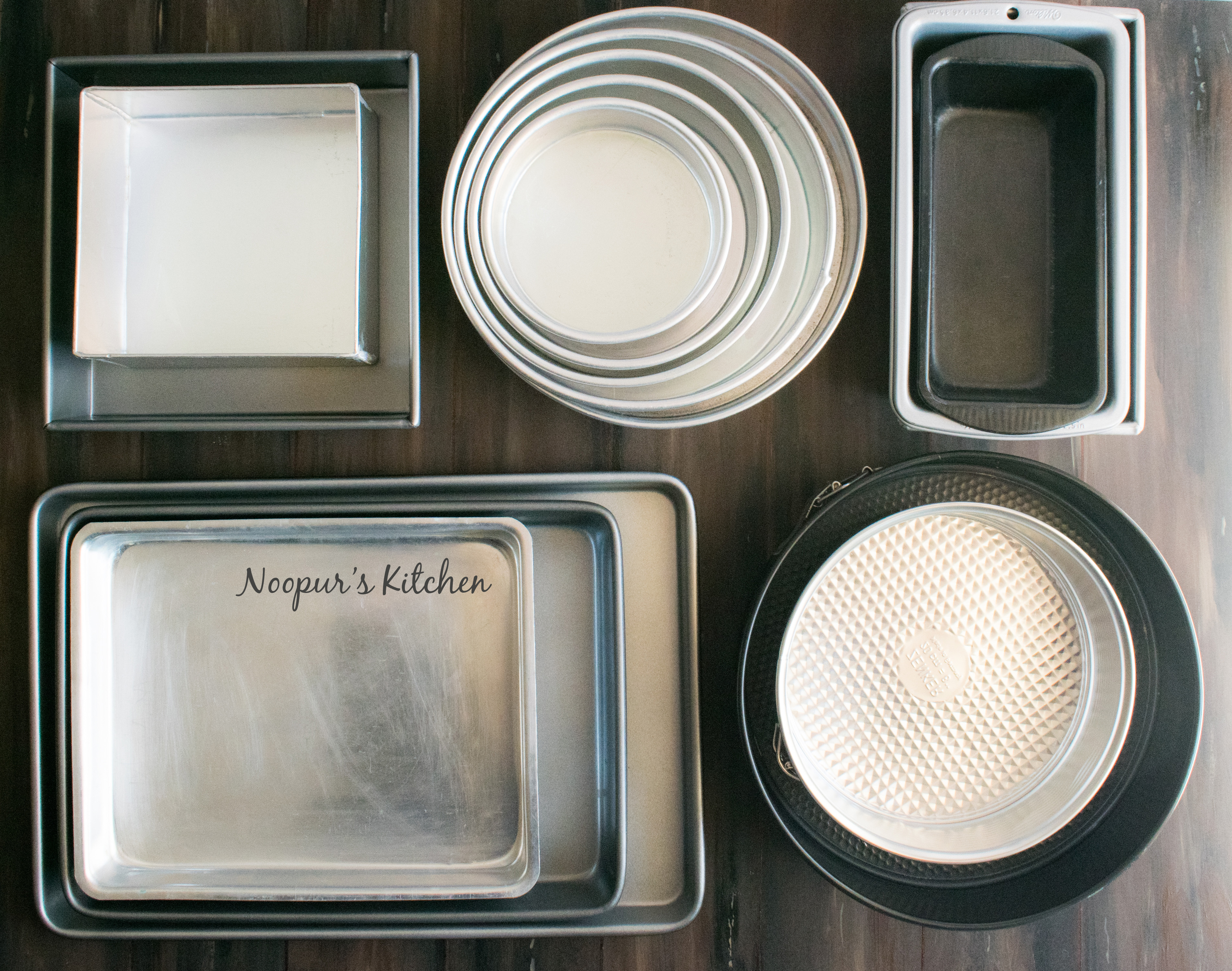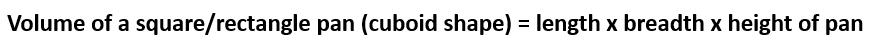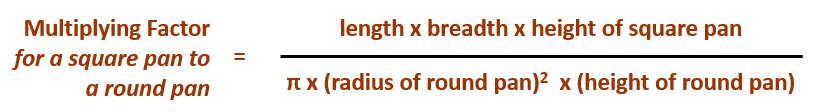# Adapting Recipes to Different Pans

Understand how to adapt a recipe to fit your pan size and shape – a simple maths and you’re ready to roll!

There are so many interesting recipes that I usually bookmark to try. Some would be fairly basic and some would require complex methods or use expensive ingredients. Most of the times if it’s a new skill or some ingredient that I haven’t worked with before, I make a small batch first. Only if all goes well and it turns out great, I’ll make it the normal size intended by the recipe publisher and it’ll go on my blog to share with all of you. Also, sometimes you need to make larger quantities for occasions than your regular recipe dictates.

Hence, it becomes important to adapt the recipe quantities as per your baking requirement.These little mathematics formulae help me convert recipes into different pan sizes & shapes easily and I thought I would share it with you today 🙂 Simply put, it’s comparing volumes of one pan to anotherIf you divide the volume of the new pan by the volume of the old pan, you’ll get the “multiplying factor”You can apply this universal formula to convert one pan to another pan (any shape or size or height) by calculating the multiplying factor by dividing their volumes. Multiply all ingredient quantities with this factor to get new quantities.

So let’s calculate the most common recipe adaptation – one round pan to another round pan.

##### Calculating Volume of Round Pans –

A round pan is actually a cylinder-shaped object geometrically.The usual height of round pans is 2″ and π ∼ 3.14. For example, the volume of an 8″ round pan would be 3.14 x 4 x 4 x 2 = 100.5 cubic inches. The volume of an 9″ round pan would be 3.14 x 4.5 x 4.5 x 2 = 127.2 cubic inches. So if you want to convert a recipe from 8″ pan to 9″ pan, you calculate the multiplying factor = 127.2/100.5 which is about 1.26. That means you’ve to increase all the quantities or recipe by 25% to get a 9″ cake.

The simplified formula (for same height pans) hence becomes –Example from 9″ pan to 7″ pan, factor = (3.5 x 3.5) / (4.5 x 4.5) = 0.6 (you can halve the recipe).

P.S. If your round pans have different heights, factor it in when calculating volumes. In case your new pan is 3″ and the old pan is 2″, multiply the factor by 1.5. If it’s the other way round, multiply the factor by 0.6.

##### Calculating volume of Square & Rectangle Pans –

A square/rectangle pan is actually a cuboid-shaped object.The usual height of these pans is 2″. For example, the volume of an 8″ square pan would be 8 x 8 x 2 = 128 cubic inches. The volume of a 13″x9″ pan would be 13 x 9 x 2 =  234 cubic inches. So if you want to convert a recipe from 8″ square pan to 13″x9″ pan, you calculate the multiplying factor = 234/128 which is about 1.8. You can double the recipe of 8″ square pan to get the quantities for a 13″x9″ pan.

##### Adapting a recipe from a square to round pan and vice versa –

Simply put,When it comes to a loaf pan, assuming the edges are straight, the volume will be still same as a cuboid. Example, the standard loaf pan is 9″ x 5″ x 2½” and hence its volume would be 112.5 cubic inches. So converting a recipe from 8″ round pan to a standard loaf pan, multiplying factor would be 112.5/100.5 = 1.1, which means the recipes can be easily swapped between these two pans (the time to bake in a loaf pan is more than a round pan).

Some basics to remember –

1. It’s really important here that you weigh your ingredients and use grams/ounces for your calculations. Use this conversion chart for easy reference.
2. Some ingredients quantities are really less. For them, you can round them off to nearest tablespoon or teaspoon measurements for ease. Examples – baking powder, baking soda, vanilla extract and salt.
3. What to do when it comes to eggs? If you have to, for example, half the 3 large eggs used in the recipe, you can either beat the eggs and use half the quantity by weight (use the rest for your omelette 🙂 ), or you replace them with 2 regular eggs.
4. This maths, in the end, is just a guideline – the pans should not be filled more than 2/3rds of its height (you can make cupcakes out of the extra batter!). Also, the bigger pans might take longer to bake than what the recipe says (and smaller might take little less), so adjust the time accordingly.

• The rules of checking if the cake is done still remain the same irrespective of the time – a toothpick inserted in centre should come out clean. (A great tool to use here is the probe cake tester, its long and gives clearer view of progress and easy to clean as well)

Now you know how to adapt a recipe to fit your pan size and shape. Once you understand the mathematics behind it, you don’t have to remember the formulae actually. You can just take out your calculator and divide the volume of the new pan by old pan and get your factor. This works for different height pans too. Use some basic intelligence to round off numbers wherever possible to make life easier.

Happy baking 🙂

P.S. – Post may contain some affiliate links